### Co-ordinate Geometry Class 9th Mathematics AP Board Solution

##### Question 1.Plot the following points in the Cartesian plane whose x, y co-ordinates are given.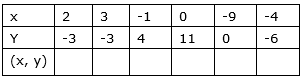Answer: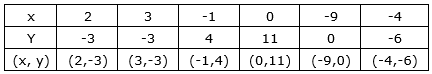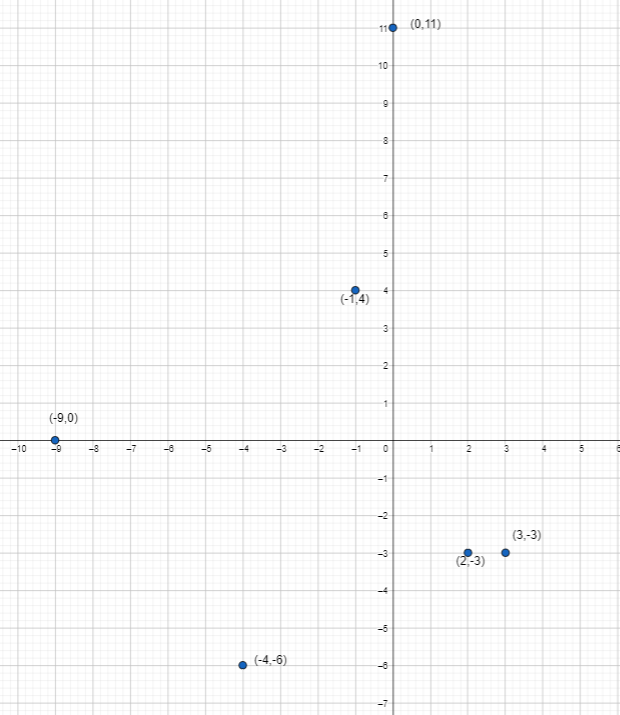Question 2.Are the positions of (5, -8) and (-8, 5) is same? Justify your answer.Answer: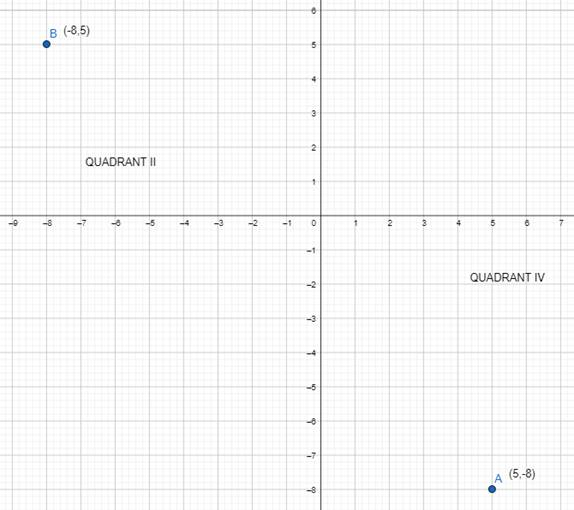No, positions of (5, -8) and (-8, 5) is not same because (5, -8) lies in IV quadrant and (-8, 5) lies in II quadrant.Question 3.What can you say about the position of the points (1, 2), (1, 3), (1, -4), (1, 0), and(1, 8). Locate on a graph sheet.Answer:From the given points (1, 2), (1, 3), (1, -4), (1, 0), and(1, 8), we can say that all these points lie on a line parallel to Y-axis at a distance of 1 unit.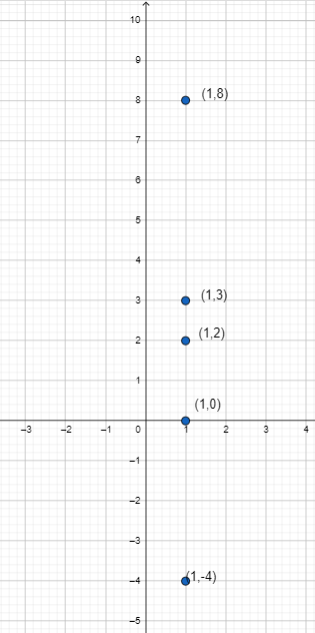Question 4.What can you say about the position of the points (5, 4), (8, 4), (3, 4), (0, 4),(-4, 4), (-2, 4)? Locate the points on a graph sheet and justify your answer.Answer:From the given points (5, 4), (8, 4), (3, 4), (0, 4),(-4, 4), (-2, 4), we can say that all these points lie on a line parallel to X-axis at a distance of 4 units.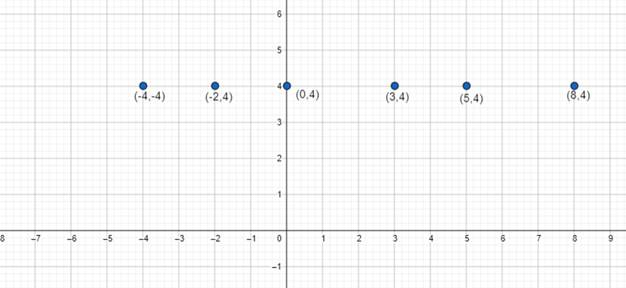Question 5.Plot the points (0, 0),(0, 3), (3, 4), (4, 0) in graph sheet. Join the points with straight lines to make a rectangle. Find the area of the rectangle.Answer:Plotting the points :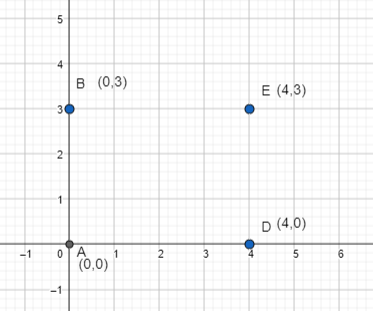Joining the lines to form a rectangle and finding its area :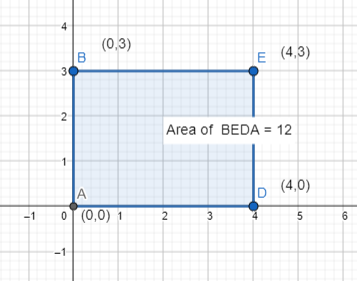Finding the area :From the graph, we can see that,L = AD = 4 unitsB = ED = 3 unitsNow, area of rectangle = L × B = 4 × 3 = 12 sq. unit.∴ Area of rectangle BEDA = 12 sq. unit.Question 6.Plot the points (2, 3), (6, 3) and (4, 7) in a graph sheet. Join them to make it a triangle. Find the area of the triangle.Answer:Plotting the points :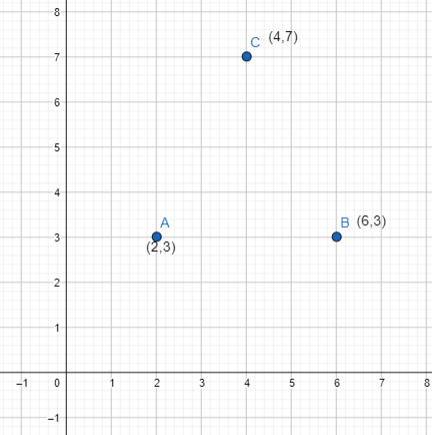Joining the points to form a triangle and finding its area :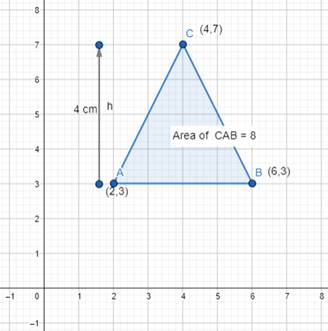Finding the area :From the graph, we can see that,H = 4 unitsB = AB = 4 unitsArea of the triangle =× B × H=× 4 × 4 = 8 sq. unit.∴ Area of triangle CAB = 8 sq. unit.Question 7.Plot at least six points in a graph sheet, each having the sum of its coordinates equal to 5. {Hint : ( -2, 7) (1, 4) .............}Answer:The points whose sum of co-ordinates is equal to 5 are :(2,3), (3,2), (0,5), (5,0), (1,4), (4,1).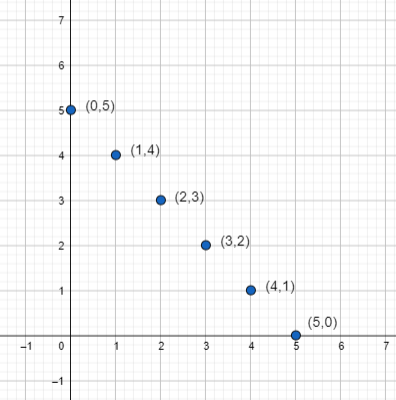Question 8.Look at the graph. Write the coordinates of the points A, B, C, D, E, F, G, H, I, J, K, L,L, M, N, P, O and Q.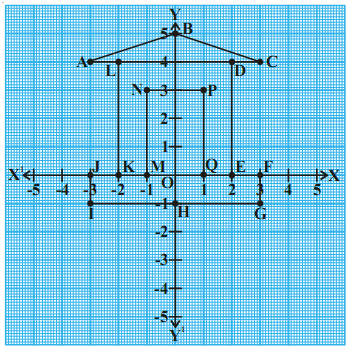Answer:From the graph, we can see that co-ordinates of the given points are :Co-ordinates of point A are : (-3,4)Co-ordinates of point B are : (0,5)Co-ordinates of point C are : (3,4)Co-ordinates of point D are : (2,4)Co-ordinates of point E are : (2,0)Co-ordinates of point F are : (3,0)Co-ordinates of point G are : (3,-1)Co-ordinates of point H are : (0,-1)Co-ordinates of point I are : (-3,-1)Co-ordinates of point J are : (-3,0)Co-ordinates of point K are : (-2,0)Co-ordinates of point L are : (-2,4)Co-ordinates of point M are : (-1,0)Co-ordinates of point N are : (-1,3)Co-ordinates of point O are : (0,0)Co-ordinates of point P are : (1,3)Co-ordinates of point Q are : (1,0)Question 9.In a graph Sheet Plot each pair of points, join them by line segmentsi. (2, 5), (4, 7)ii. (-3, 5), (-1, 7)iii. (-3, -4), (2, -4)iv. (-3, -5), (2, -5)v. (4, -2), (4, -3)vi. (-2, 4), (-2, 3)vii. (-2, 1), (-2, 0)Now join the following pairs of points by straight line segments, in the same graph.viii. (-3, 5), (-3, 4)ix. (2, 5), (2, -4)x. (2, -4), (4, -2)xi. (2, -4), (4, -3)xii. (4, -2), (4, 7)xiii. (4, 7), (-1, 7)xiv. (-3, 2), (2, 2)Now you will get a surprise figure.What is it?Answer:Plotting the first set of points and forming their line segments :i. (2, 5), (4, 7) → A,Bii. (-3, 5), (-1, 7) → C,Diii. (-3, -4), (2, -4) → E,Fiv. (-3, -5), (2, -5) → G,Hv. (4, -2), (4, -3) → I,Jvi. (-2, 4), (-2, 3) → K,Lvii. (-2, 1), (-2, 0) → M,N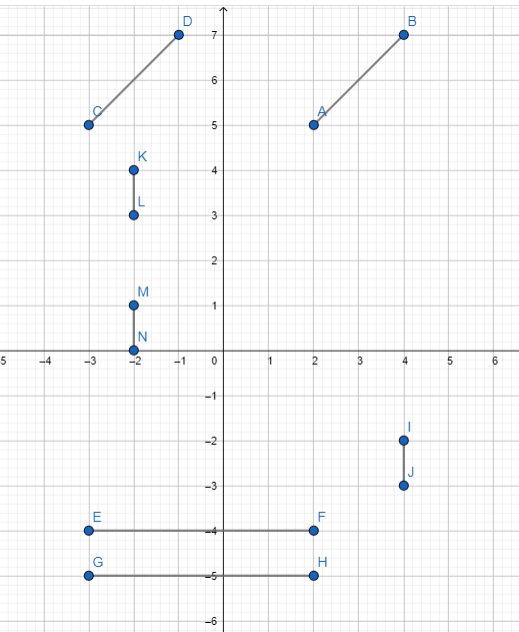Plotting the second set of points and forming their line segments :viii. (-3, 5), (-3, 4) → O,Pix. (2, 5), (2, -4) → Q,Rx. (2, -4), (4, -2) →S,Txi. (2, -4), (4, -3) → U,Vxii. (4, -2), (4, 7) → W,Xxiii. (4, 7), (-1, 7) →Y,Zxiv. (-3, 2), (2, 2) → A1,B1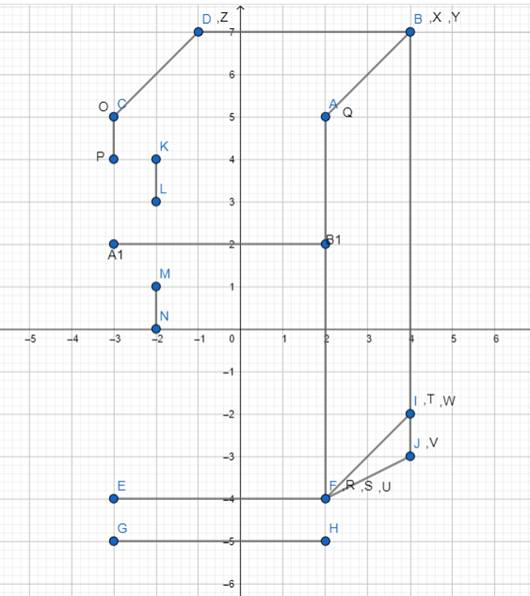PDF FILE TO YOUR EMAIL IMMEDIATELY PURCHASE NOTES & PAPER SOLUTION. @ Rs. 50/- each (GST extra)

HINDI ENTIRE PAPER SOLUTION

MARATHI PAPER SOLUTION

SSC MATHS I PAPER SOLUTION

SSC MATHS II PAPER SOLUTION

SSC SCIENCE I PAPER SOLUTION

SSC SCIENCE II PAPER SOLUTION

SSC ENGLISH PAPER SOLUTION

SSC & HSC ENGLISH WRITING SKILL

HSC ACCOUNTS NOTES

HSC OCM NOTES

HSC ECONOMICS NOTES

HSC SECRETARIAL PRACTICE NOTES

# 2019 Board Paper Solution

HSC ENGLISH SET A 2019 21st February, 2019

HSC ENGLISH SET B 2019 21st February, 2019

HSC ENGLISH SET C 2019 21st February, 2019

HSC ENGLISH SET D 2019 21st February, 2019

SECRETARIAL PRACTICE (S.P) 2019 25th February, 2019

HSC XII PHYSICS 2019 25th February, 2019

CHEMISTRY XII HSC SOLUTION 27th, February, 2019

OCM PAPER SOLUTION 2019 27th, February, 2019

HSC MATHS PAPER SOLUTION COMMERCE, 2nd March, 2019

HSC MATHS PAPER SOLUTION SCIENCE 2nd, March, 2019

SSC ENGLISH STD 10 5TH MARCH, 2019.

HSC XII ACCOUNTS 2019 6th March, 2019

HSC XII BIOLOGY 2019 6TH March, 2019

HSC XII ECONOMICS 9Th March 2019

SSC Maths I March 2019 Solution 10th Standard11th, March, 2019

SSC MATHS II MARCH 2019 SOLUTION 10TH STD.13th March, 2019

SSC SCIENCE I MARCH 2019 SOLUTION 10TH STD. 15th March, 2019.

SSC SCIENCE II MARCH 2019 SOLUTION 10TH STD. 18th March, 2019.

SSC SOCIAL SCIENCE I MARCH 2019 SOLUTION20th March, 2019

SSC SOCIAL SCIENCE II MARCH 2019 SOLUTION, 22nd March, 2019

XII CBSE - BOARD - MARCH - 2019 ENGLISH - QP + SOLUTIONS, 2nd March, 2019

# HSCMaharashtraBoardPapers2020

(Std 12th English Medium)

HSC ECONOMICS MARCH 2020

HSC OCM MARCH 2020

HSC ACCOUNTS MARCH 2020

HSC S.P. MARCH 2020

HSC ENGLISH MARCH 2020

HSC HINDI MARCH 2020

HSC MARATHI MARCH 2020

HSC MATHS MARCH 2020

# SSCMaharashtraBoardPapers2020

(Std 10th English Medium)

English MARCH 2020

HindI MARCH 2020

Hindi (Composite) MARCH 2020

Marathi MARCH 2020

Mathematics (Paper 1) MARCH 2020

Mathematics (Paper 2) MARCH 2020

Sanskrit MARCH 2020

Sanskrit (Composite) MARCH 2020

Science (Paper 1) MARCH 2020

Science (Paper 2)

Geography Model Set 1 2020-2021

MUST REMEMBER THINGS on the day of Exam

Are you prepared? for English Grammar in Board Exam.

Paper Presentation In Board Exam

How to Score Good Marks in SSC Board Exams

Tips To Score More Than 90% Marks In 12th Board Exam

How to write English exams?

How to prepare for board exam when less time is left

How to memorise what you learn for board exam

No. 1 Simple Hack, you can try out, in preparing for Board Exam

How to Study for CBSE Class 10 Board Exams Subject Wise Tips?

JEE Main 2020 Registration Process – Exam Pattern & Important Dates

NEET UG 2020 Registration Process Exam Pattern & Important Dates

How can One Prepare for two Competitive Exams at the same time?

8 Proven Tips to Handle Anxiety before Exams!